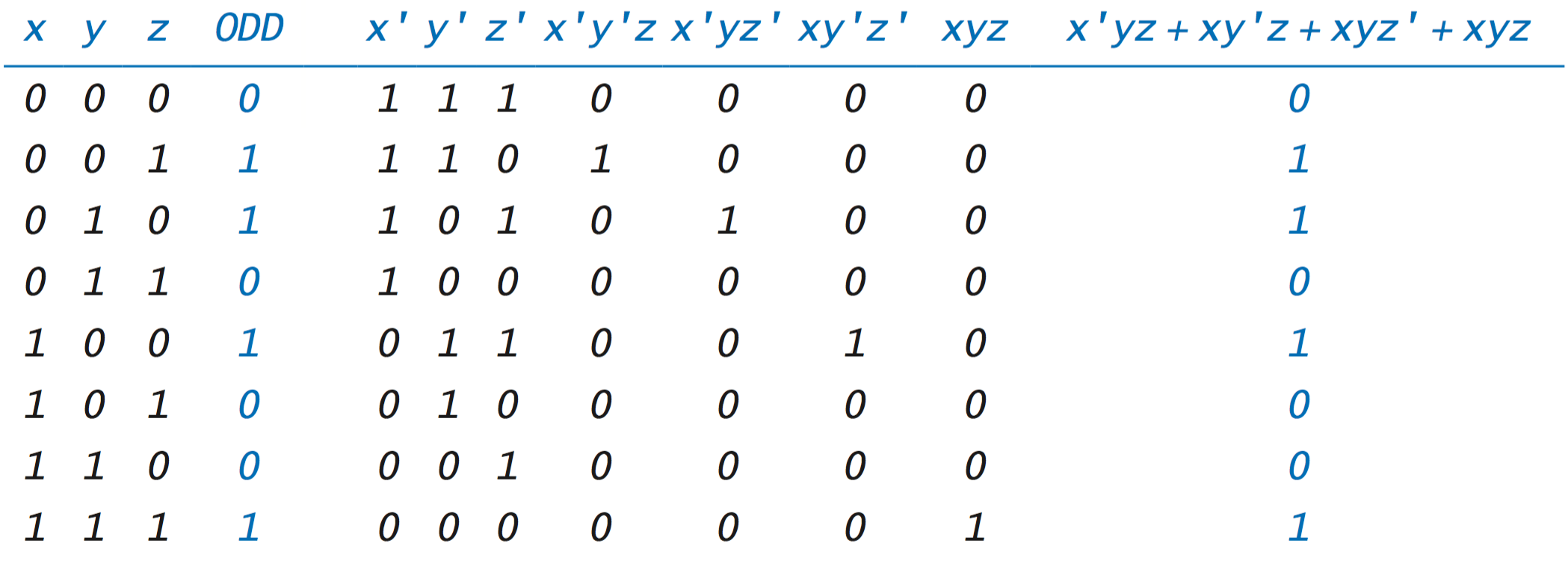# 7.1   Boolean Logic

A boolean function is a mathematical function that maps arguments to a value, where the allowable values of range (the function arguments) and domain (the function value) are just one of two values— true and false (or 0 and 1). The study of boolean functions is known as Boolean logic.

## Boolean functions.

To define any boolean function, we need only to specify its value for each possible value of its inputs. The not function is a boolean function of one variable.
\quad\quad\quad\quad\quad\quad \begin{align} NOT(x) &\;=\; \begin{cases} 1 & \text {if x is 0} \\[1ex] 0 & \text {if x is 1} \end{cases} \end{align}
The and, or, and exclusive or functions are familiar boolean functions of two variables.
\quad\quad\quad\quad\quad\quad \begin{align} AND(x, y) &\;=\; \begin{cases} 1 & \text {if both x and y are 1} \\[1ex] 0 & \text {otherwise} \end{cases} \\ \\ OR(x, y) &\;=\; \begin{cases} 1 & \text {if either x or y (or both) is 1} \\[1ex] 0 & \text {otherwise} \end{cases} \\ \\ XOR(x, y) &\;=\; \begin{cases} 1 & \text {if x and y are different} \\[1ex] 0 & \text {otherwise} \end{cases} \end{align}
• Notation. There are many competing notations for elementary boolean functions. In this chapter, we primarily use the circuit-design notation.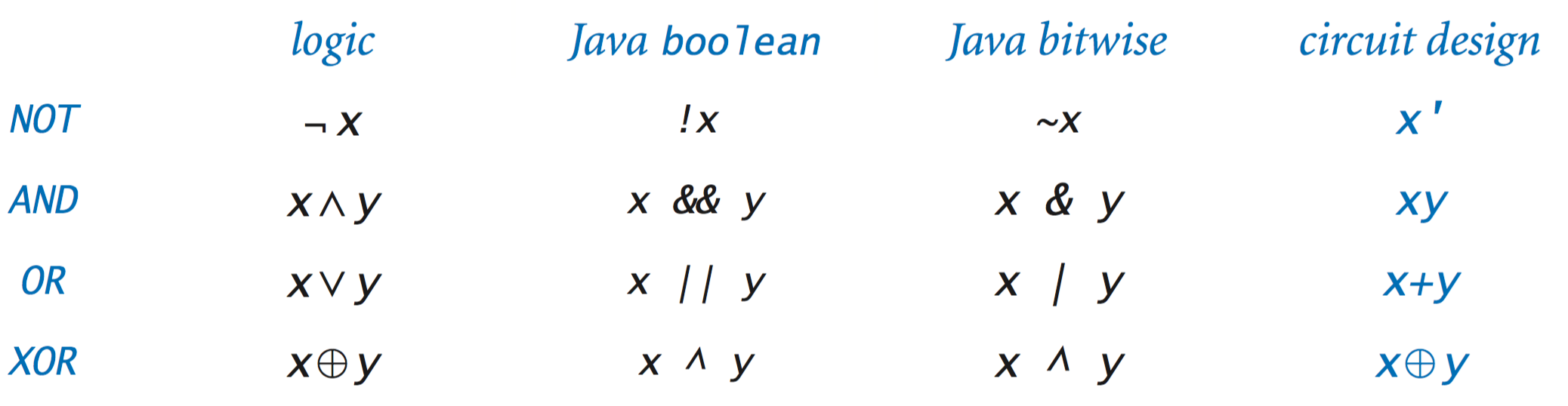• Truth tables. One way to define a boolean function is to specify its value for each possible value of its arguments. We use a truth table to do so in an organized way. A truth table has one column for each variable, one row for each possible combination of variable values, and a column that specifies the value of the function for that combination.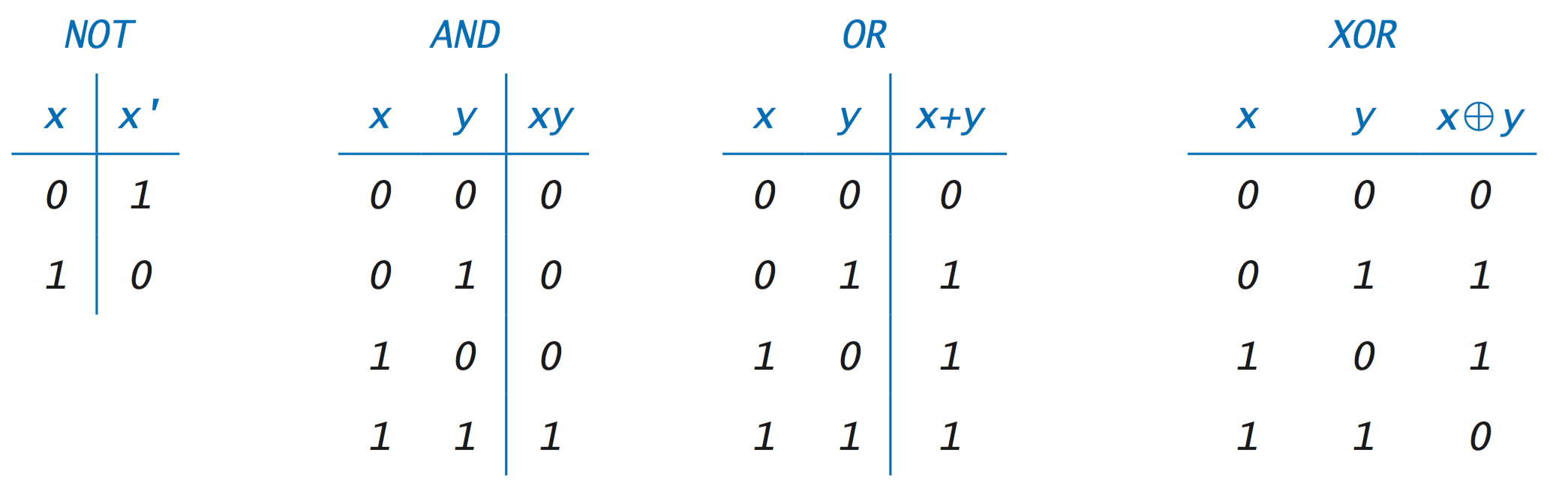A truth table for a function of n variables has 2n rows.
• Boolean algebra. Boolean algebra refers to symbolic manipulation of expressions made up of boolean variables and boolean operators. The familiar identity, commutative, distributive, and associative axioms from algebra define the axioms of Boolean algebra, along with the two complementary axioms.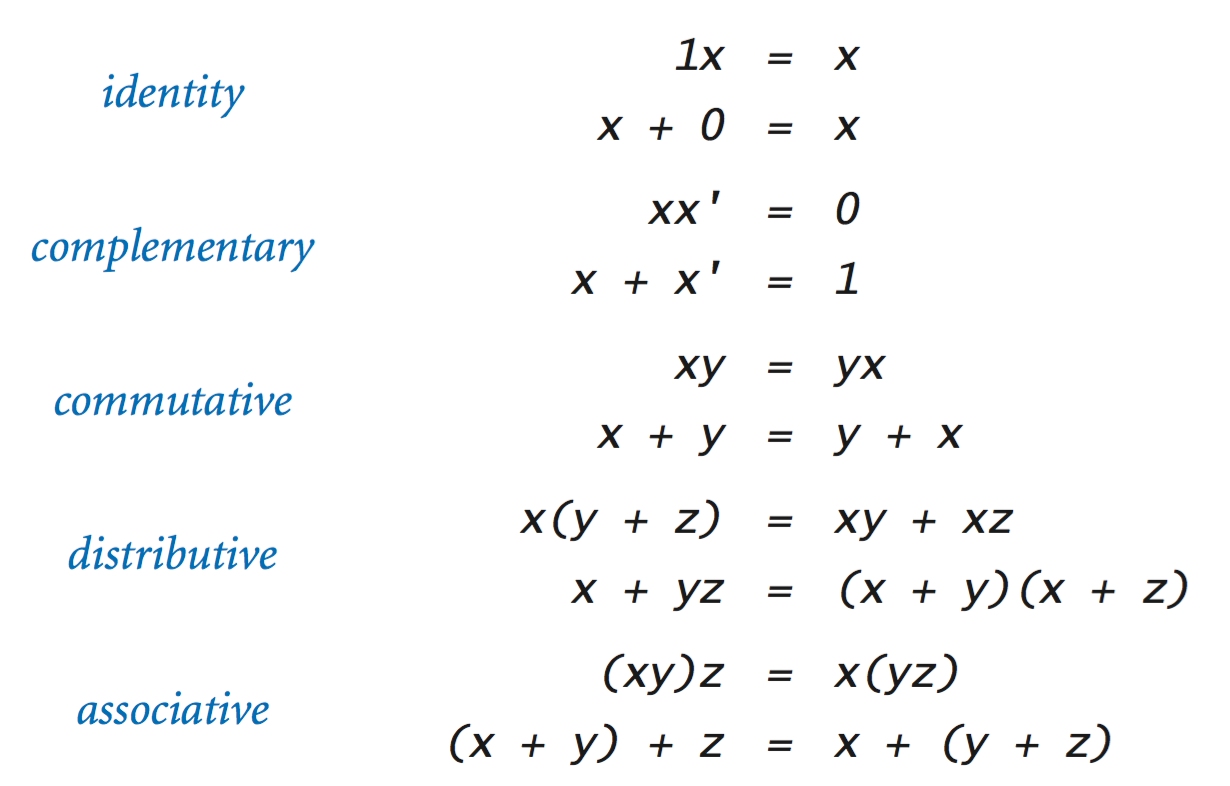In addition, you can derive many other laws from these axioms. For example, the last entry in the table gives two special identities known as DeMorgan’s laws.• Boolean algebra in Java. You can incorporate Boolean algebra into your Java programs, in two different ways.
• Java’s boolean data type: In Section 1.2, we introduced boolean operations with the values true and false and the AND, OR, and NOT operations using the operators &&, ||, and !, respectively.
• Bitwise operations on integer values: In Section 6.1, we discussed Java’s bitwise operations, which use the AND, OR, NOT, and XOR operators on each bit in the binary representations of integer values, using the operators &, |, ~, and ^, respectively.

## Boolean functions of three or more variables.

As the number of variables increases, the number of possible functions increases dramatically. There are 28 different boolean functions of 3 variables, 216 functions of 4 variables, 232 functions of 5 variables, and so forth. Several such functions play a critical role in computation and in circuit design, so we will now consider them.
• AND and OR functions. The definitions of the AND and OR functions for multiple arguments generalize in a natural way from our two-argument definitions:
\quad\quad\quad\quad\quad\quad \begin{align} AND(x_1, x_2, \ldots, x_n) &\;=\; \begin{cases} 1 & \text {if all arguments are 1} \\[1ex] 0 & \text {otherwise} \end{cases} \\ \\ OR(x_1, x_2, \ldots, x_n) &\;=\; \begin{cases} 1 & \text {if any argument is 1} \\[1ex] 0 & \text {otherwise} \end{cases} \\ \end{align}
• Majority and odd-parity functions. We consider two additional functions that arise in the design of digital circuits: the majority and odd-parity functions:
\quad\quad\quad\quad\quad\quad \begin{align} MAJ(x_1, x_2, \ldots, x_n) &\;=\; \begin{cases} 1 & \text {if strictly more arguments are 1 than 0} \\[1ex] 0 & \text {otherwise} \end{cases} \\ \\ ODD(x_1, x_2, \ldots, x_n) &\;=\; \begin{cases} 1 & \text {if an odd number of arguments are 1} \\[1ex] 0 & \text {otherwise} \end{cases} \\ \end{align}
• Boolean expressions. As with boolean functions of two variables, we can use a truth table to explicitly specify a boolean function.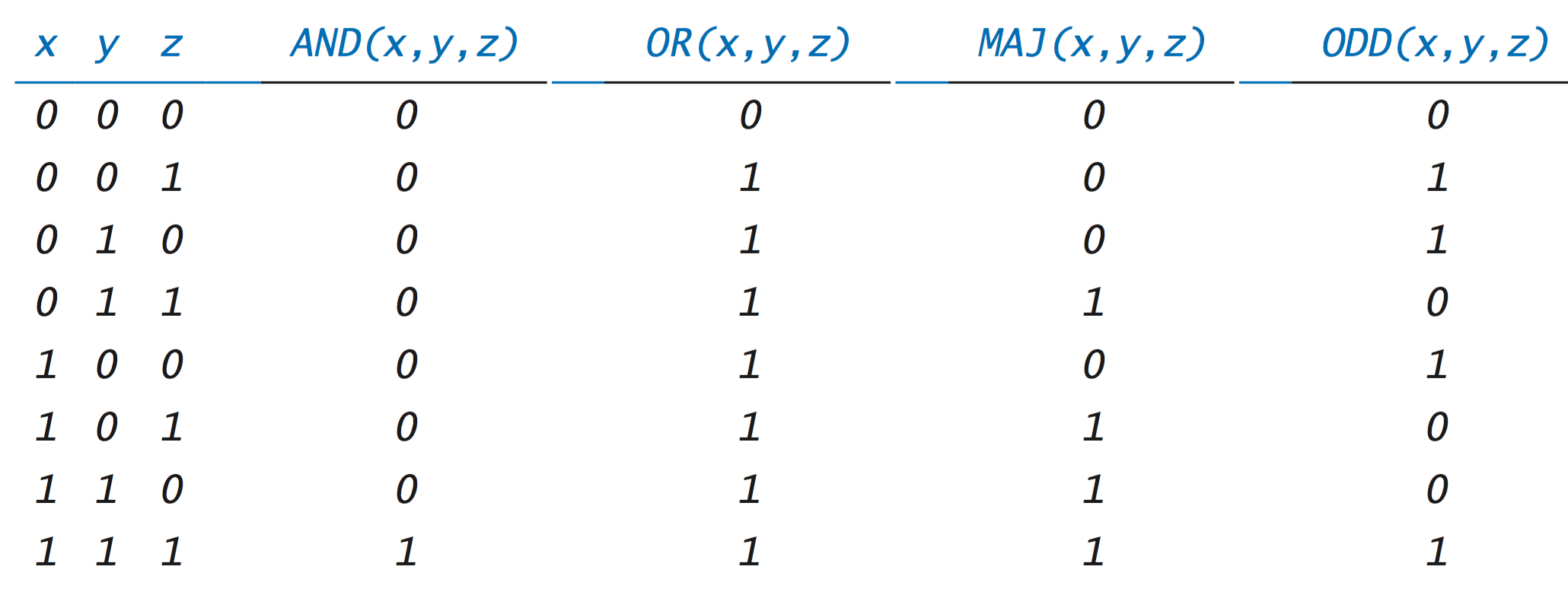This representation is cumbersome and quickly fails for functions with larger numbers of variables, since the number of rows needed for n variables is 2n. Instead, we often prefer to use boolean expressions to define boolean functions. For example, it is not hard to verify these two identities:
\quad\quad\quad\quad\quad\quad \begin{align} AND(x_1, x_2, \ldots, x_n) &\;=\; x_1 x_2 \ldots x_n \\ \\ OR(x_1, x_2, \ldots, x_n) &\;=\; x_1 + x_2 + \ldots + x_n \end{align}
• Sum-of-products representations. One of the fundamental results of Boolean algebra is that every boolean function can be represented with an expression that uses AND, OR, and NOT operators and no others. For example, consider the following truth table: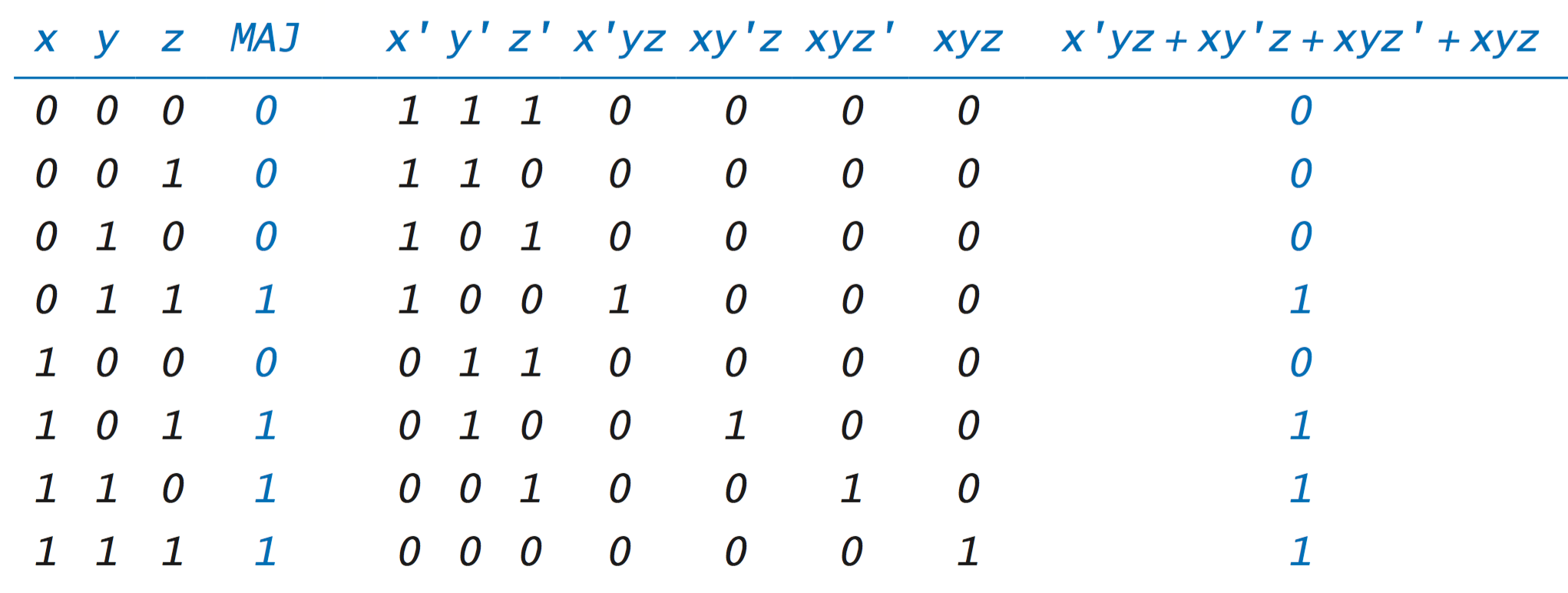Since their entries are equal for every value of the variables, the two columns highlighted in blue represent a proof of the following equation:
$$\quad\quad\quad\quad\quad\quad MAJ(x, y, z) = x'yz + xy'z + xyz' + xyz$$
We can derive such an expression for any boolean function from its truth table: For each of the rows in the truth table in which the function value is 1, we create a term that is 1 if the input variables have the values on that row and 0 otherwise. Each term is the product of each input variable (if its corresponding entry on the row in question is 1) or its negation (if the entry is 0). The sum of all of these terms gives back the function.

The boolean expression that we construct is known as the sum-of-products representation or the disjunctive normal form of the function. As another example, here is the table for the odd parity function: